## Case Studies - Electric Power: Questions

The Case Studies - Electric Power Concept Builder is comprised of 36 questions. The questions are divided into 18 different Question Groups. There are two questions in each Question Group; questions in the same group are rather similar to one another. The Concept Builder is coded to select at random a question from each group until a student is successful with that group of questions.

This Concept Builder consists of three different difficulty levels. Those three activities are described as follows:

• Apprentice Difficulty Level: Question Groups 1-6. Two circuits are given; there is only one stated difference between the two circuits. Students must determine in which circuit the light bulb is brightest.
• Master Difficulty Level: Question Groups 7-12. Two circuits are given; there are two stated differences between the two circuits. Students must determine in which circuit the light bulb is brightest.
• Wizard Difficulty Level: Question Groups 13-18. Two circuits are given; there are two stated differences between the two circuits. Students must determine in which circuit the power is greatest and by what factor it is greaterst..

The questions from each group are shown below. Teachers are encouraged to view the questions in order to judge which activities are most appropriate for their classes. We recommend providing students two or more options.

The Physics Classroom grants teachers and other users the right to print these questions for private use. Users are also granted the right to copy the text and modify it for their own use. However, this document should not be uploaded to other servers for distribution to and/or display by others. The Physics Classroom website should remain the only website or server from which the document is distributed or displayed. We also provide a PDF that teachers can use under the same conditions. We have included a link to the PDF near the bottom of this page.

#### Case Studies - Electric Power

Apprentice Difficulty Level
Question Group 1
Question 1
Circuit A and B have the same current. But the light bulb in Circuit A has twice the resistance of the light bulb in Circuit B. In which circuit will the bulb be the brightest?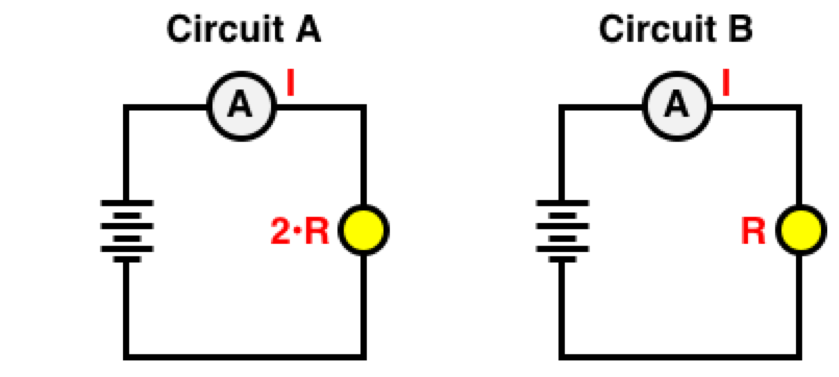Question 2
Circuit A and B have the same current. But the light bulb in Circuit A has one-half the resistance of the light bulb in Circuit B. In which circuit will the bulb be the brightest?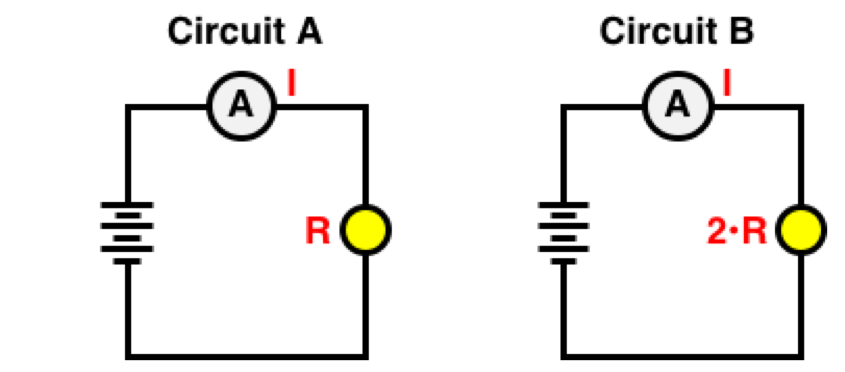Question Group 2
Question 3
The light bulbs in Circuit A and B have the same resistance. But the current in Circuit A is two times the current in Circuit B. In which circuit will the bulb be the brightest?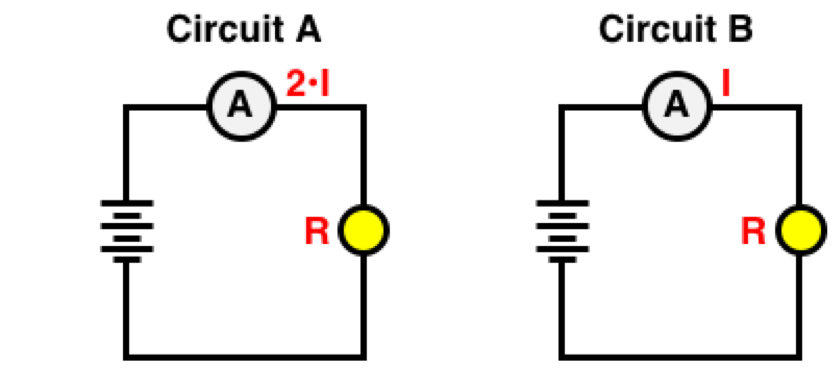Question 4
The light bulbs in Circuit A and B have the same resistance. But the current in Circuit A is one-half the current in Circuit B. In which circuit will the bulb be the brightest?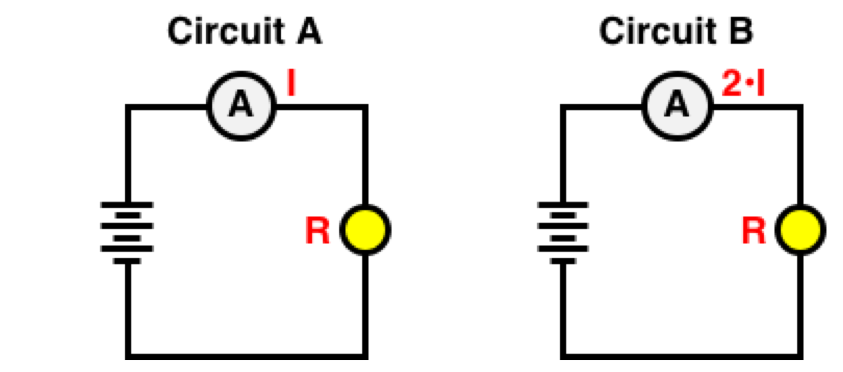Question Group 3
Question 5
Circuit A and B have the same current. But the battery of Circuit A has twice the voltage of the battery in Circuit B. In which circuit will the bulb be the brightest?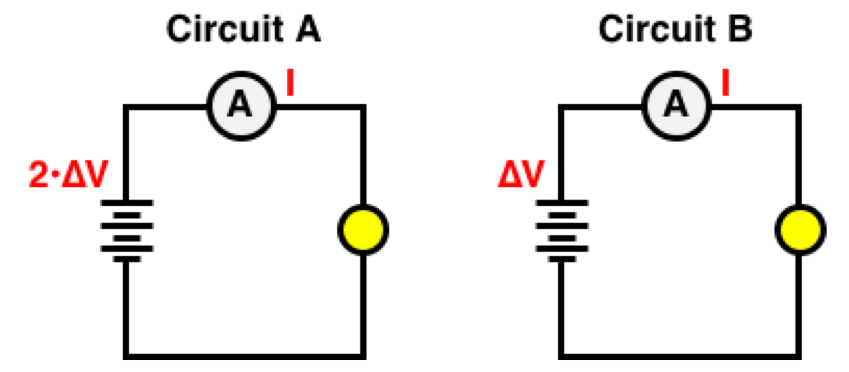Question 6
Circuit A and B have the same current. But the battery of Circuit A has one-half the voltage of the battery in Circuit B. In which circuit will the bulb be the brightest?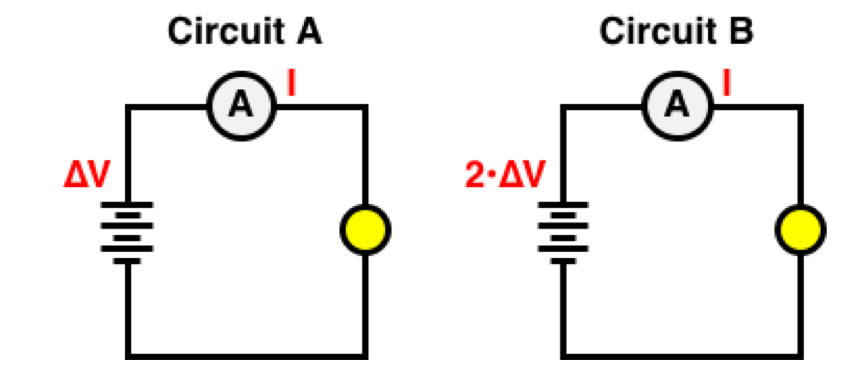Question Group 4
Question 7
Circuit A and B have the same battery voltage. But the current in Circuit A is two times the current in Circuit B. In which circuit will the bulb be the brightest?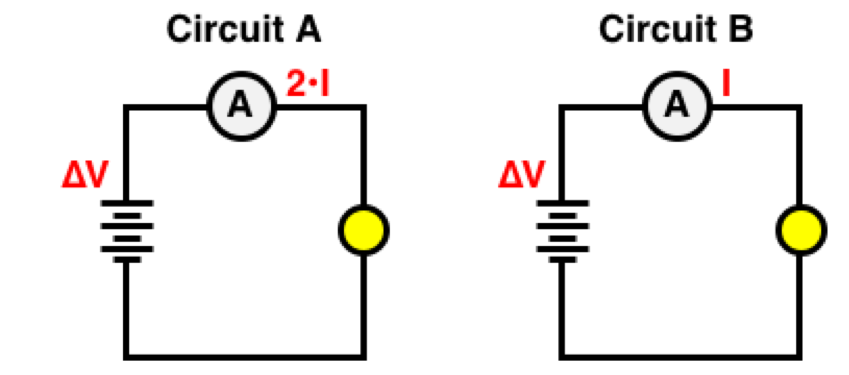Question 8
Circuit A and B have the same battery voltage. But the current in Circuit A is one-half the current in Circuit B. In which circuit will the bulb be the brightest?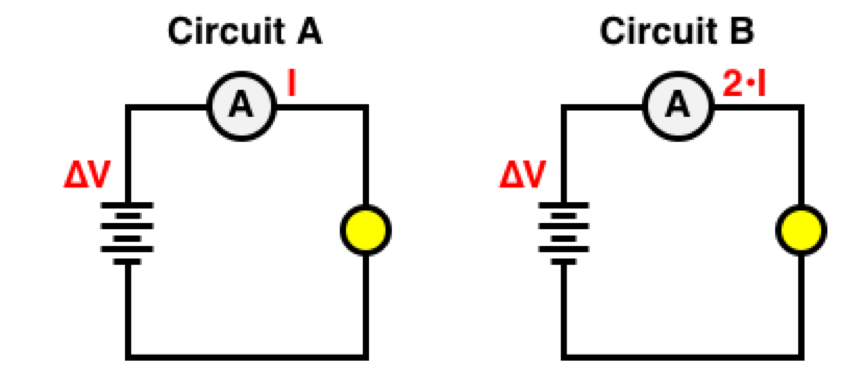Question Group 5
Question 9
The bulbs in Circuit A and B have the same resistance. But the battery of Circuit A has twice the voltage as the battery of Circuit B. In which circuit will the bulb be the brightest?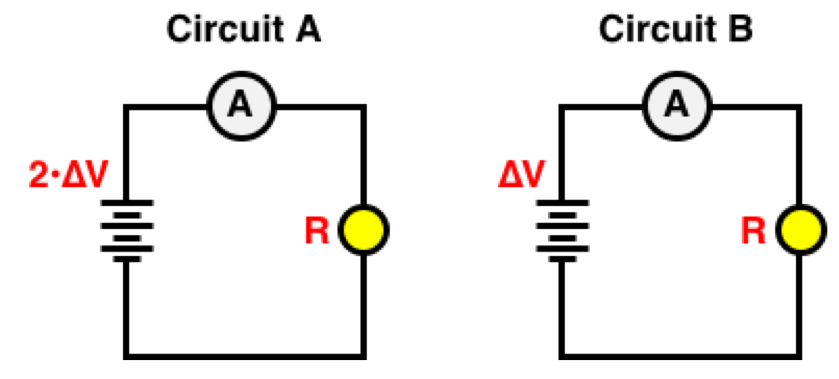Question 10
The bulbs in Circuit A and B have the same resistance. But the battery of Circuit A has one-half the voltage as the battery of Circuit B. In which circuit will the bulb be the brightest?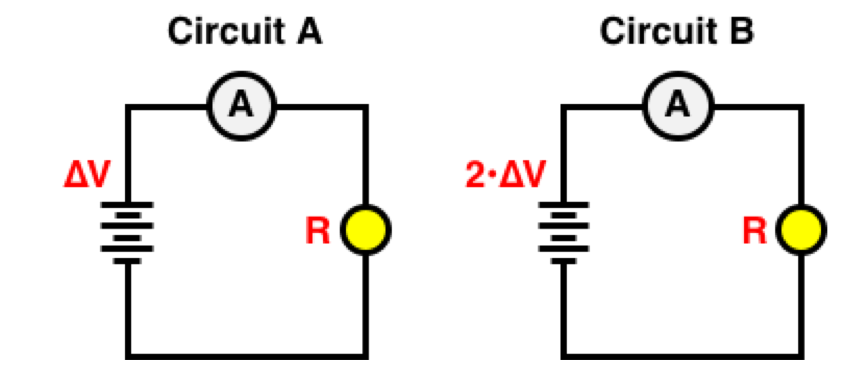Question Group 6
Question 11
Circuit A and B have the same battery voltage. But the light bulb in Circuit A has twice the resistance of the light bulb in Circuit B. In which circuit will the bulb be the brightest?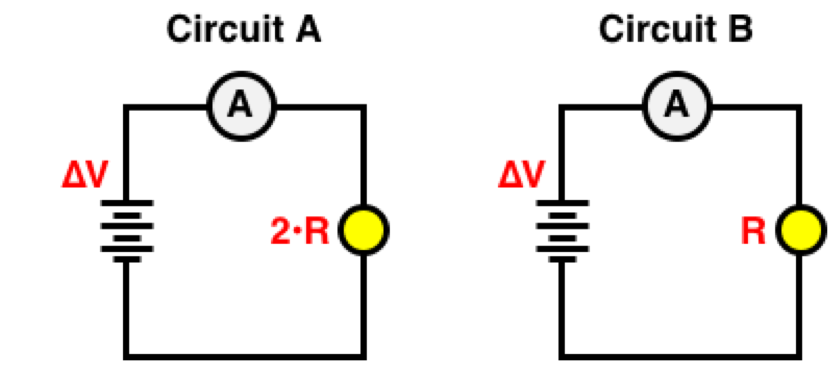Question 12
Circuit A and B have the same battery voltage. But the light bulb in Circuit A has one-half the resistance of the light bulb in Circuit B. In which circuit will the bulb be the brightest?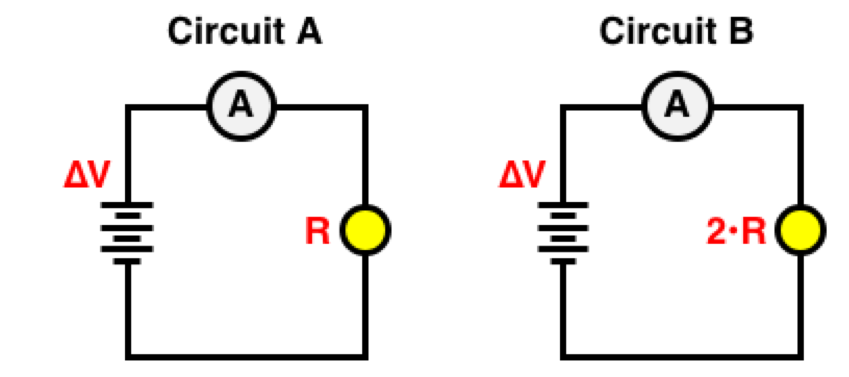Master Difficulty Level
Question Group 7
Question 13
The current in Circuit A is two times the current in Circuit B. The light bulb in Circuit A has one-half the resistance of the light bulb in Circuit B. In which circuit will the bulb be the brightest?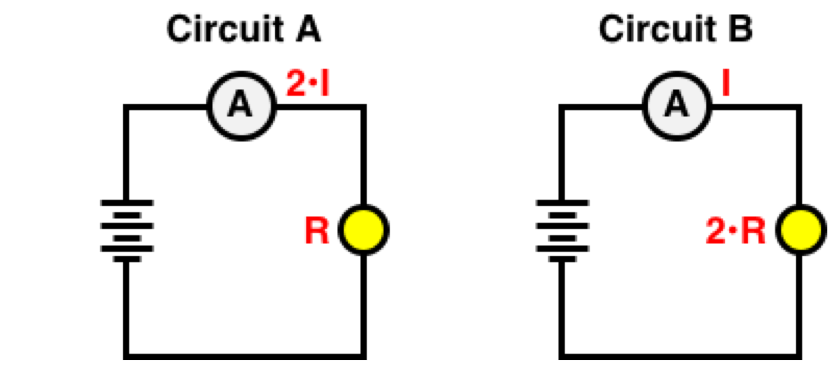Question 14
The current in Circuit A is one-half the current in Circuit B. The light bulb in Circuit A has twice the resistance of the light bulb in Circuit B. In which circuit will the bulb be the brightest?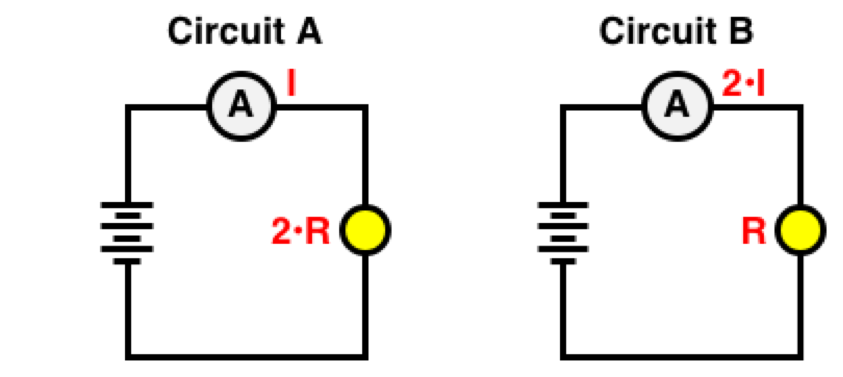Question Group 8
Question 15
The battery of Circuit A has twice the voltage as the battery of Circuit B. The light bulb in Circuit A has one-half the resistance of the light bulb in Circuit B. In which circuit will the bulb be the brightest?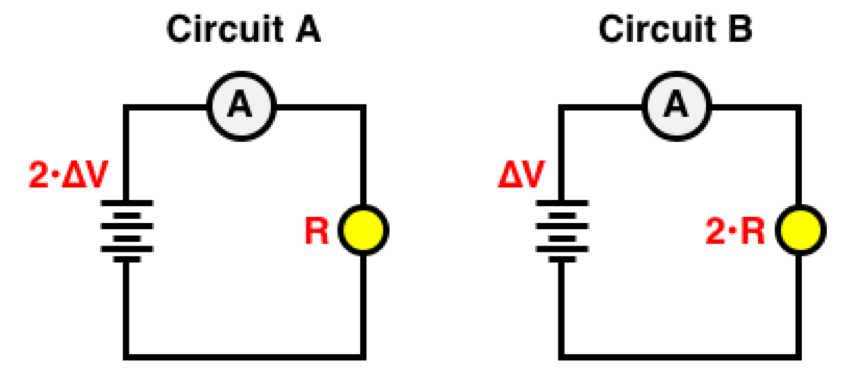Question 16
The battery of Circuit A has one-half the voltage as the battery of Circuit B. The light bulb in Circuit A has two times the resistance of the light bulb in Circuit B. In which circuit will the bulb be the brightest?Question Group 9
Question 17
The current in Circuit A is two times the current in Circuit B. The light bulb in Circuit A has two times the resistance of the light bulb in Circuit B. In which circuit will the bulb be the brightest?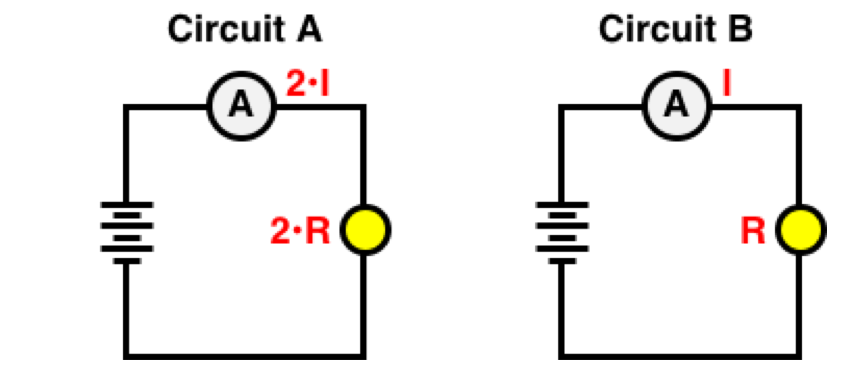Question 18
The current in Circuit A is one-half the current in Circuit B. The light bulb in Circuit A has one-half the resistance of the light bulb in Circuit B. In which circuit will the bulb be the brightest?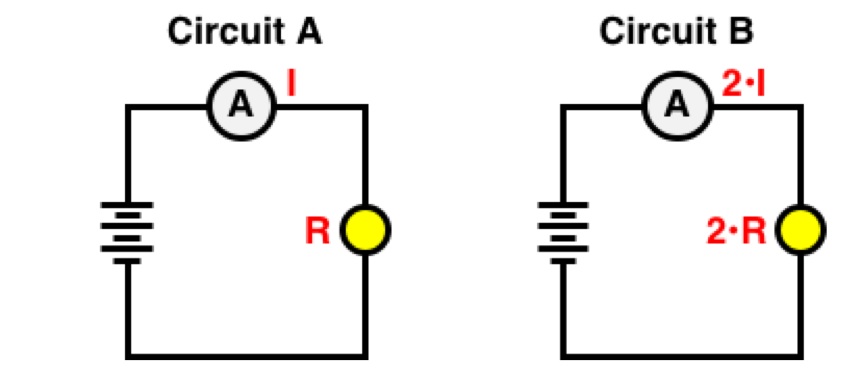Question Group 10
Question 19
The battery of Circuit A has twice the voltage of the battery in Circuit B. The light bulb in Circuit A has two times the resistance of the light bulb in Circuit B. In which circuit will the bulb be the brightest?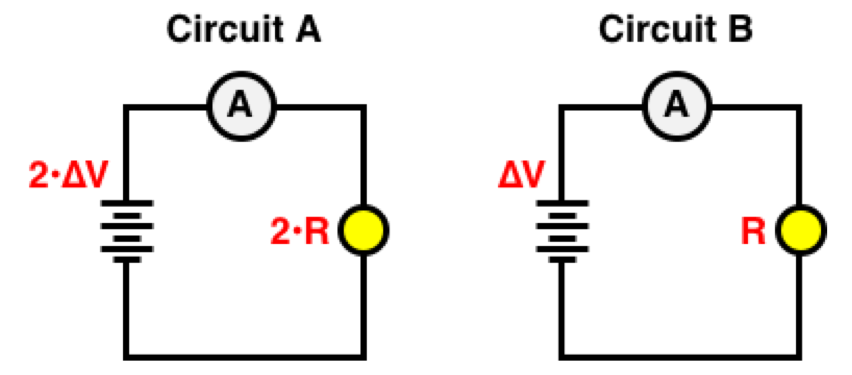Question 20
The battery of Circuit A has one-half the voltage of the battery in Circuit B. The light bulb in Circuit A has one-half the resistance of the light bulb in Circuit B. In which circuit will the bulb be the brightest?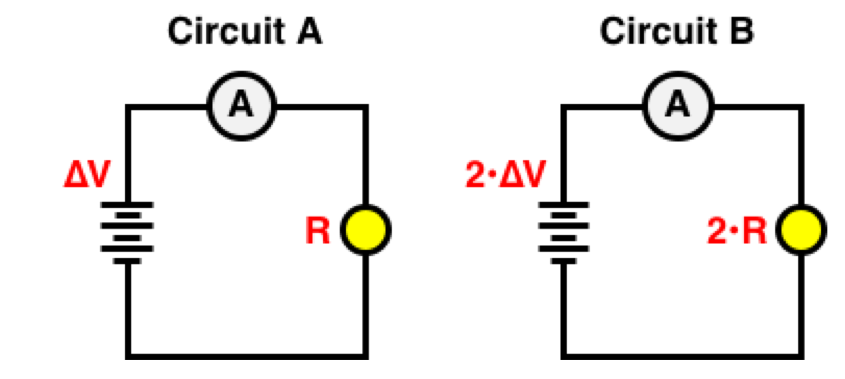Question Group 11
Question 21
The current in Circuit A is two times the current in Circuit B. The battery of Circuit A has one-half the voltage of the battery in Circuit B. In which circuit will the bulb be the brightest?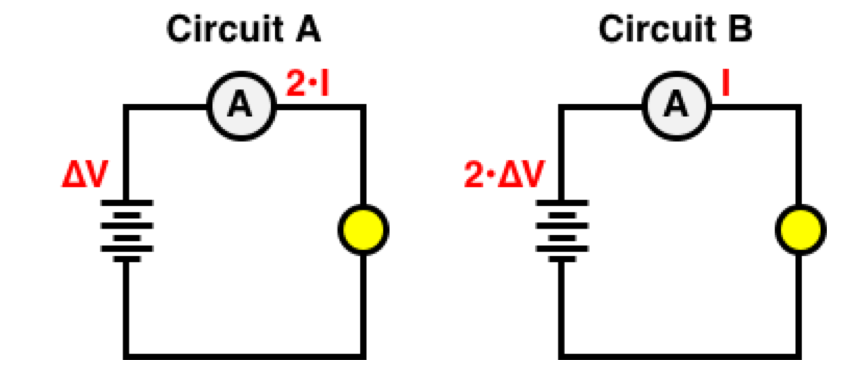Question 22
The current in Circuit A is one-half the current in Circuit B. The battery of Circuit A has two times the voltage of the battery in Circuit B. In which circuit will the bulb be the brightest?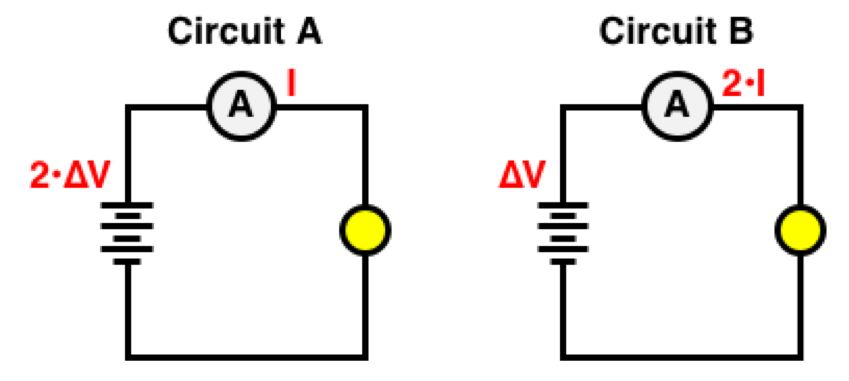Question Group 12
Question 23
The current in Circuit A is two times the current in Circuit B. The light bulb in Circuit A has one-half the resistance of the light bulb in Circuit B. In which circuit will the bulb be the brightest?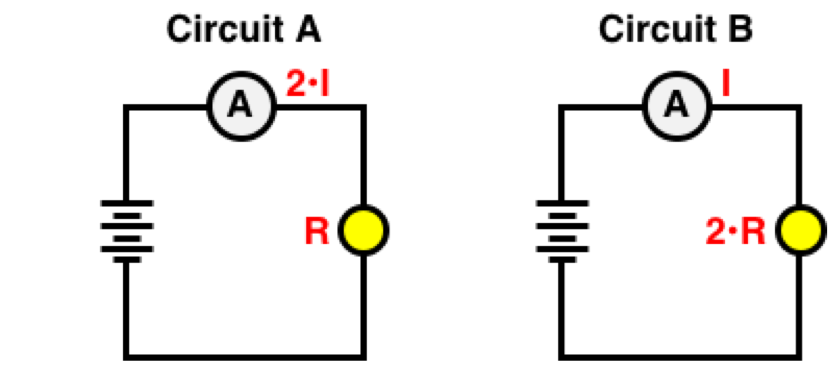Question 24
The current in Circuit A is one-half the current in Circuit B. The light bulb in Circuit A has two times the resistance of the light bulb in Circuit B. In which circuit will the bulb be the brightest?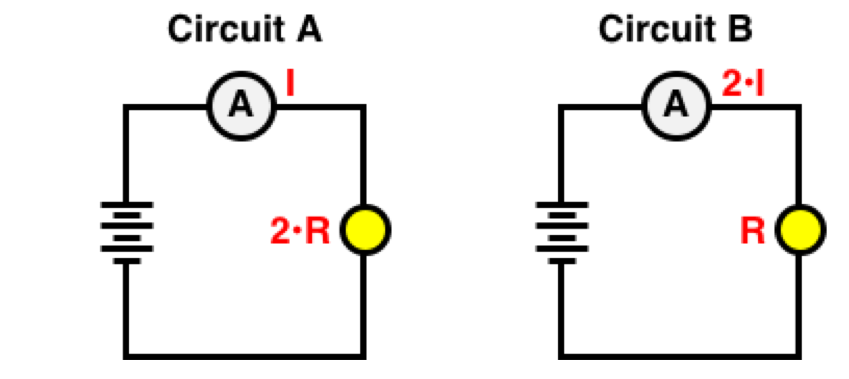Wizard Difficulty Level
Question Group 13
Question 25
The current in Circuit A is two times the current in Circuit B. The light bulb in Circuit A has one-half the resistance of the light bulb in Circuit B. Which circuit has the greatest power? ... and by what factor?Question 26
The current in Circuit A is one-half the current in Circuit B. The light bulb in Circuit A has twice the resistance of the light bulb in Circuit B. Which circuit has the greatest power? ... and by what factor?Question Group 14
Question 27
The battery of Circuit A has twice the voltage as the battery of Circuit B. The light bulb in Circuit A has one-half the resistance of the light bulb in Circuit B. Which circuit has the greatest power? ... and by what factor?Question 28
The battery of Circuit A has one-half the voltage as the battery of Circuit B. The light bulb in Circuit A has two times the resistance of the light bulb in Circuit B. Which circuit has the greatest power? ... and by what factor?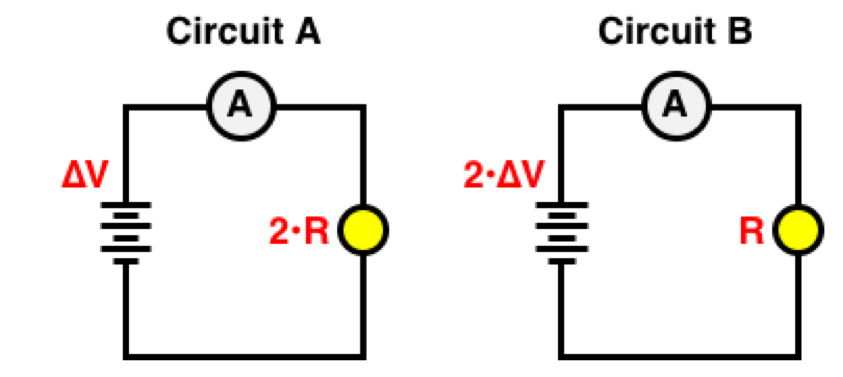Question Group 15
Question 29
The current in Circuit A is two times the current in Circuit B. The light bulb in Circuit A has two times the resistance of the light bulb in Circuit B. Which circuit has the greatest power? ... and by what factor?Question 30
The current in Circuit A is one-half the current in Circuit B. The light bulb in Circuit A has one-half the resistance of the light bulb in Circuit B. Which circuit has the greatest power? ... and by what factor?Question Group 16
Question 31
The battery of Circuit A has twice the voltage of the battery in Circuit B. The light bulb in Circuit A has two times the resistance of the light bulb in Circuit B. Which circuit has the greatest power? ... and by what factor?Question 32
The battery of Circuit A has one-half the voltage of the battery in Circuit B. The light bulb in Circuit A has one-half the resistance of the light bulb in Circuit B. Which circuit has the greatest power? ... and by what factor?Question Group 17
Question 33
The light bulbs in Circuit A and B have the same resistance. But the current in Circuit A is two times the current in Circuit B. Which circuit has the greatest power? ... and by what factor?Question 34
The light bulbs in Circuit A and B have the same resistance. But the current in Circuit A is one-half the current in Circuit B. Which circuit has the greatest power? ... and by what factor?Question Group 18
Question 35
The current in Circuit A is two times the current in Circuit B. The light bulb in Circuit A has one-half the resistance of the light bulb in Circuit B. Which circuit has the greatest power? ... and by what factor?Question 36
The current in Circuit A is one-half the current in Circuit B. The light bulb in Circuit A has two times the resistance of the light bulb in Circuit B. Which circuit has the greatest power? ... and by what factor?